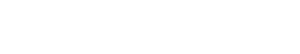# Term Frequency–Inverse Document Frequency (tf-idf) matrix

The Term Frequency–Inverse Document Frequency (tf-idf) matrix is a statistical measure used to evaluate how important a word is to a document in a corpus. The tf-idf value increases proportionally to the number of times a word appears in the document, but is offset by the frequency of the word in the corpus, which helps to adjust for the fact that some words are more common than others.

The tf-idf matrix is used as a weighting factor in information retrieval and text mining. The tf-idf matrix can be used to calculate the similarity between two documents, or to find out which document contains the most relevant information for a given query term.

## Tf-idf vs tf-idf matrix

The tf-idf matrix is a specific type of tf-idf that is used for text analytics. While the term tf-idf is commonly used in a general sense, the tf-idf matrix is a more specific statistical measure. The tf-idf matrix can be used to calculate the similarity between two documents, or to find out which document contains the most relevant information for a given query term.

## Other similar terms

There are a few other terms that are similar to Term Frequency–Inverse Document Frequency (tf-idf) matrix, but are not exactly the same thing. These terms are:

BM25: BM25 is a ranking function that is used in information retrieval. BM25 is similar to tf-idf, but it also takes into account the length of the document.

LSI: Latent Semantic Indexing (LSI) is a technique that is used to find relationships between terms in a corpus. LSI is similar to tf-idf, but it uses Singular Value Decomposition (SVD) to reduce the dimensionality of the data. There are a few other terms that are similar to Term Frequency–Inverse Document Frequency (tf-idf) matrix, but are not exactly the same thing.Unlock the power of actionable insights with AI-based natural language processing.